Equity. Access. Excellence.

# DCMP Data Analysis Tools

Explore statistical concepts in an interactive way. Use the DCMP Data Analysis Tools to construct graphs, obtain summary statistics, find probabilities under the normal distribution, get confidence intervals, fit linear regression models, and more!

The Dana Center Mathematics Pathways (DCMP) offers this suite of data analysis tools to complement the Dana Center’s Introductory Statistics: Analyzing Data with Purpose course.

Throughout this course, students analyze data, construct and test hypotheses, solve problems, reflect on their work, and make connections between concepts. Students use the DCMP Data Analysis Tools to analyze and understand data and run simulations to promote deep understanding of statistical analysis.

## Exploratory Data Analysis

• Describing and Exploring Categorical Data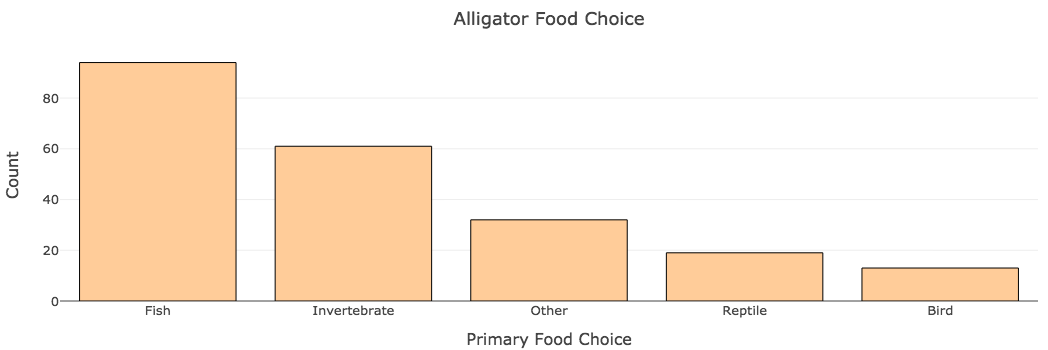Construct frequency and contingency tables and bar graphs to explore distributions of categorical variables. For one or two categorical variables.

• Describing and Exploring Quantitative VariablesFind summary statistics and construct interactive histograms, boxplots, dotplots, or stem-and leaf plots. For one, two, or more groups.

• Explore Time Series Data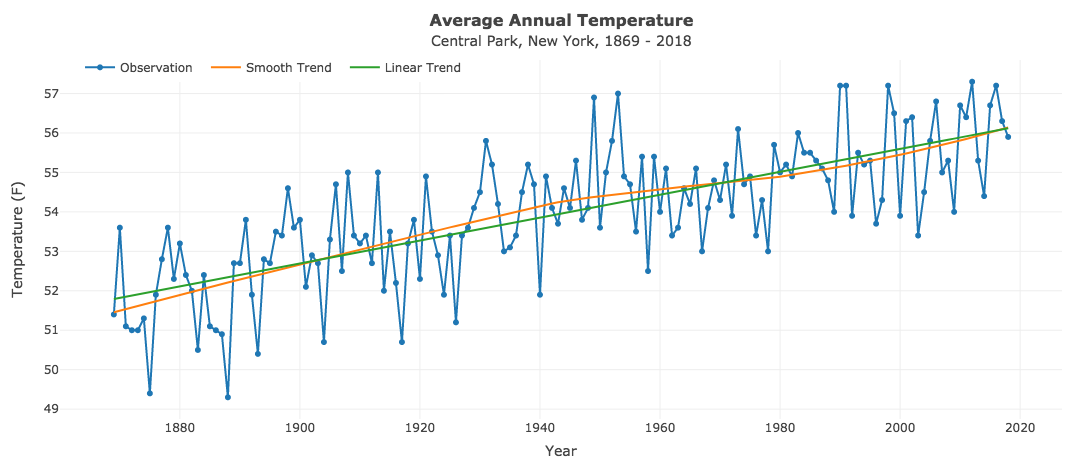Plot a simple time series and add a smooth or linear trend. Use preloaded data or provide your own.

• Mean vs. Median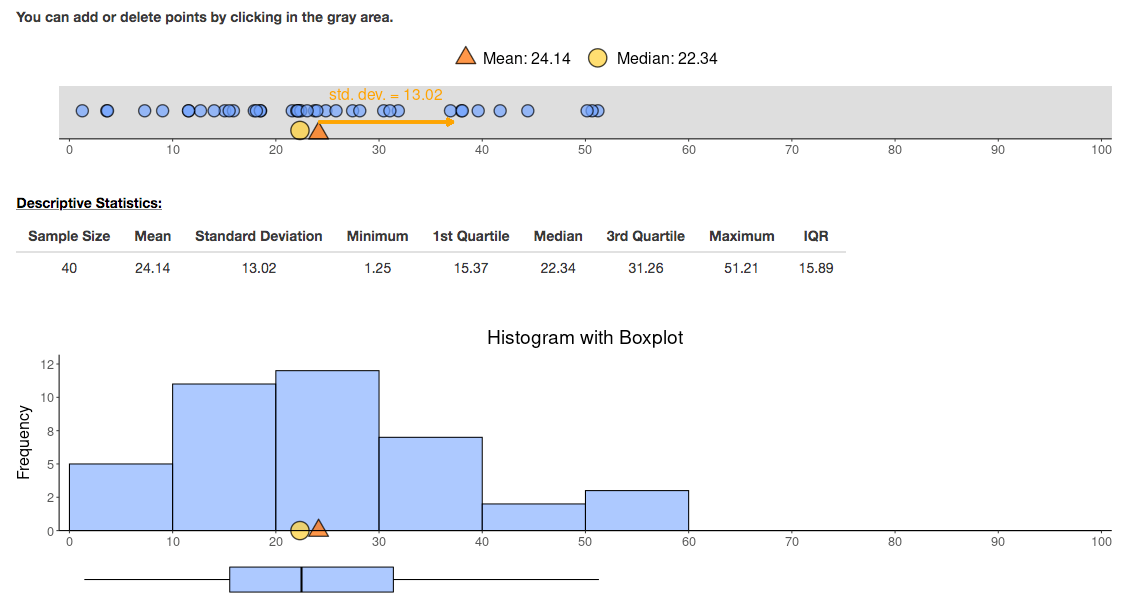Explore the relationship between the mean and median for data derived from a variety of distributions or enter your own data.

• Generate Random Numbers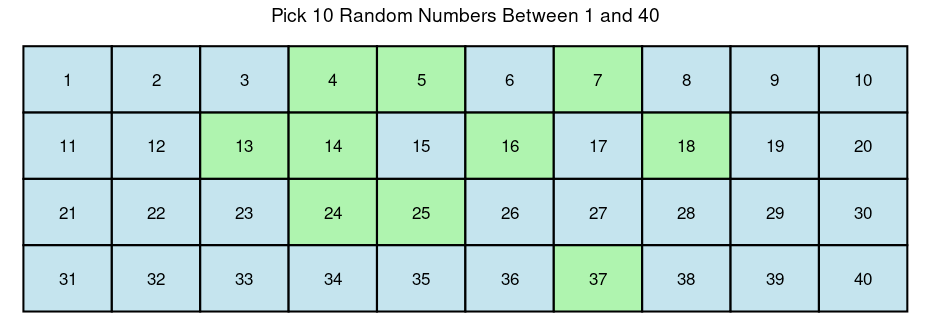Generate random numbers or flips of a (biased) coin. Keep track of generated numbers with a bar chart.

## Association, Correlation, and Regression

• Association Between Two Categorical Variables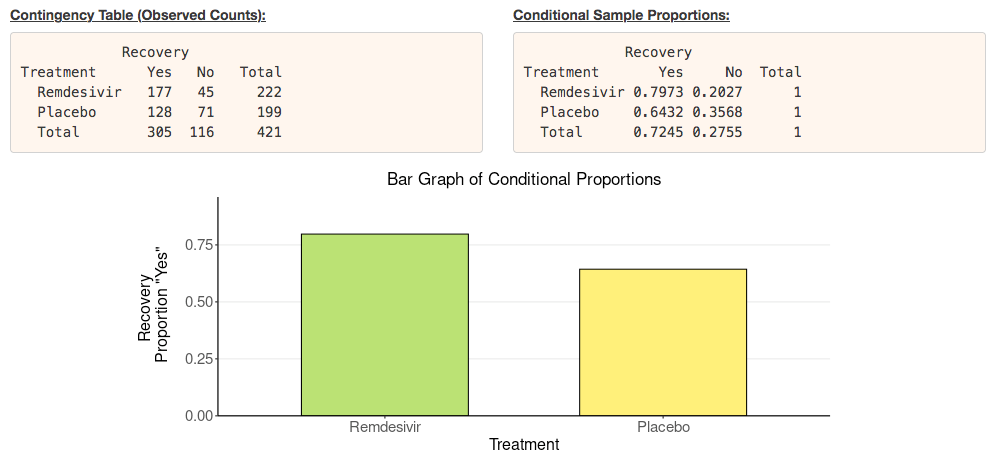Construct 2 x 2 contingency tables, obtain conditional proportions, and get a bar graph. Find the difference or ratio of proportions to describe the strength of the association. Build the sampling distribution of the difference or ratio via resampling.

• Relationship Between Two Quantitative Variables: The Correlation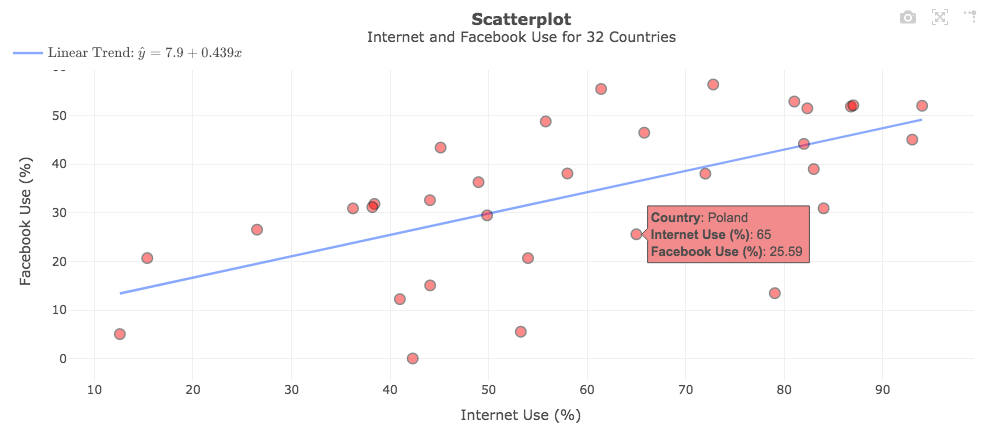Construct interactive scatterplots, hover over points, move or remove points, and overlay a smooth trend line. Find the correlation coefficient r and see if it is robust to outliers. Build the sampling distribution of r via resampling.

• Correlation Game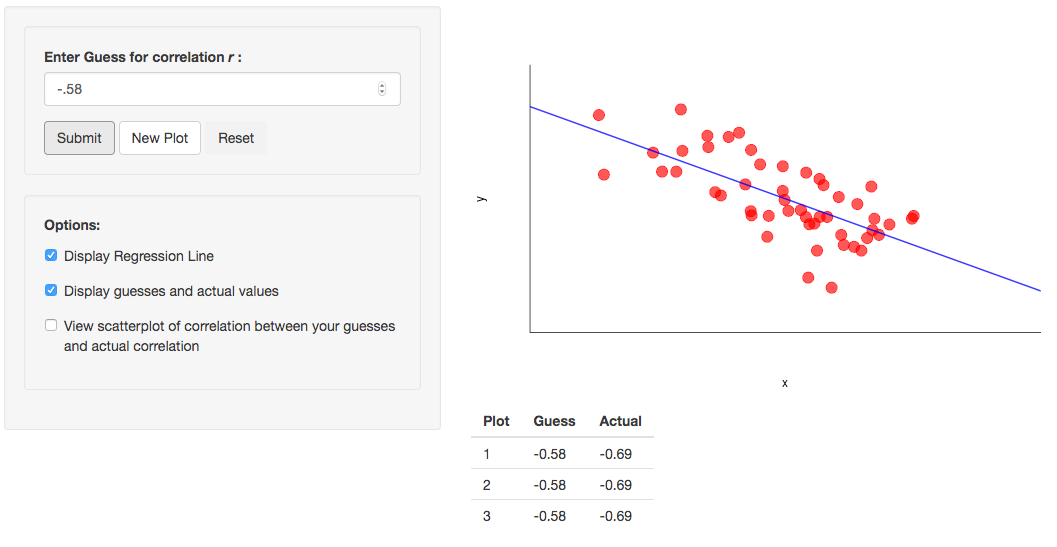Randomly generate scatterplots to guess the correlation coefficient r. Optionally, display the regression line. See how your guesses correlate with the actual values.

• Explore Linear Regression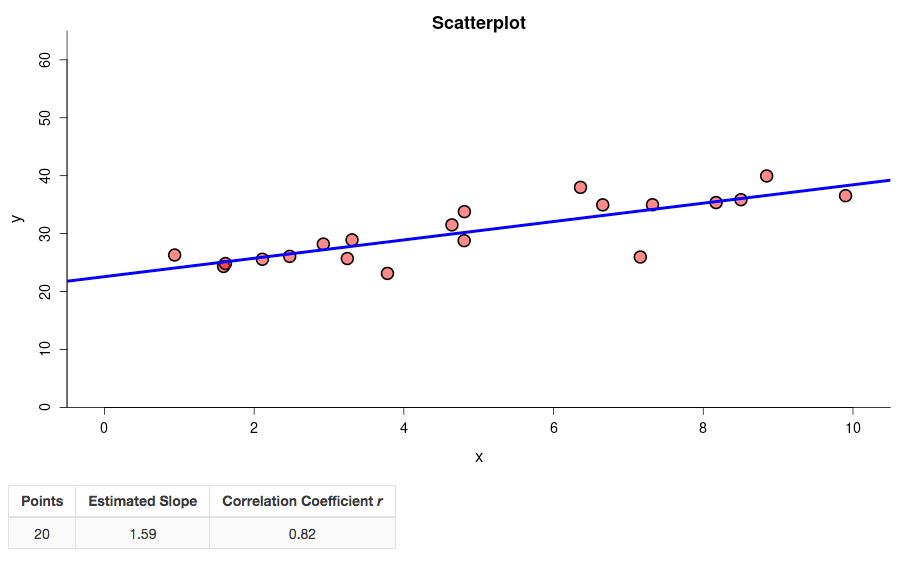Create scatterplots from scratch by clicking in an empty plot to add or remove points. Investigate the effect of outliers on the correlation coefficient or regression line. Simulate linear or non-linear relationships.

• Linear Regression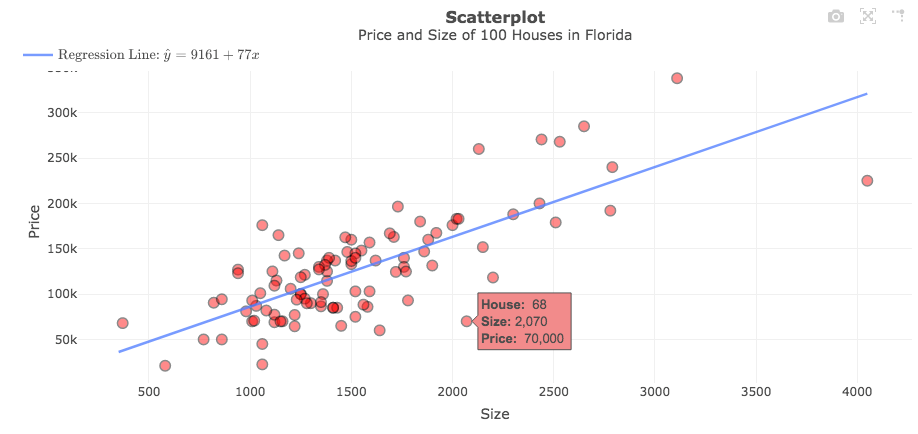Fit a simple linear regression model and obtain the regression equation and related statistics such as r-squared. Make predictions and construct confidence intervals. Display and analyze residuals.

• Exploring Multivariate Relationships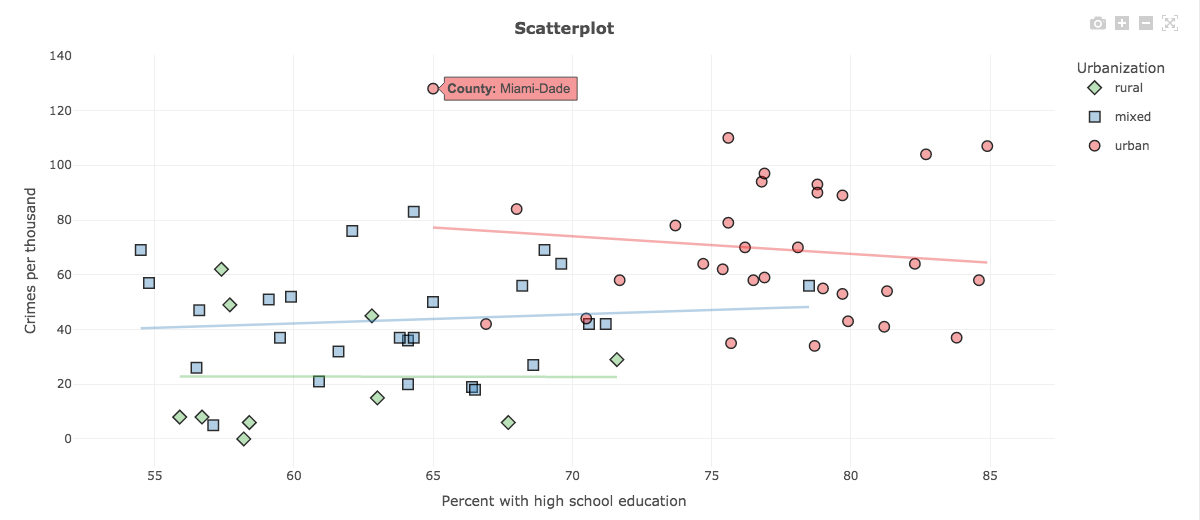Construct interactive scatterplots to explore the relationship between two quantitative variables while accounting for a third (categorical or quantitative) grouping variable. Fit bivariate multiple linear regression models.

## Distributions

• The Normal Distribution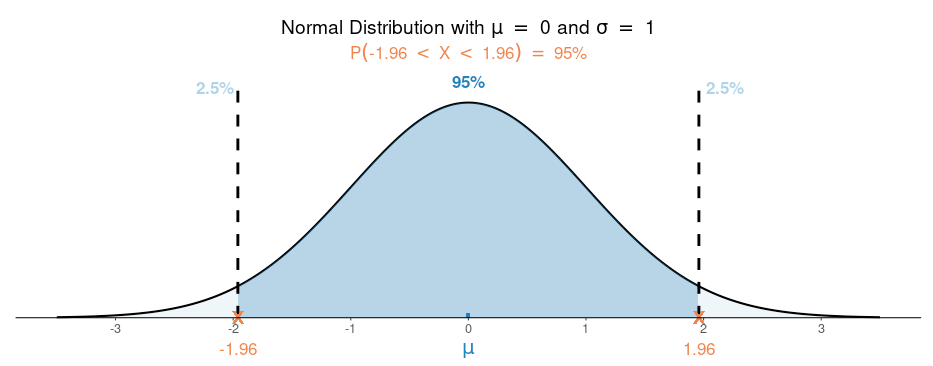See how the shape of the normal distribution depends on the mean and standard deviation. Find and visualize one- and two-tailed probabilities and percentiles (critical values).

• The t Distribution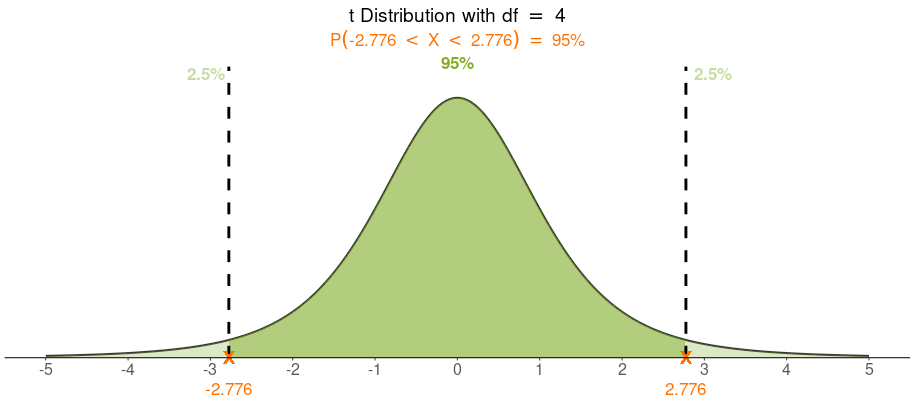See how the shape of the t distribution depends on the degrees of freedom. Find and visualize one- and two-tailed probabilities and percentiles (critical values).

• The Chi-Squared Distribution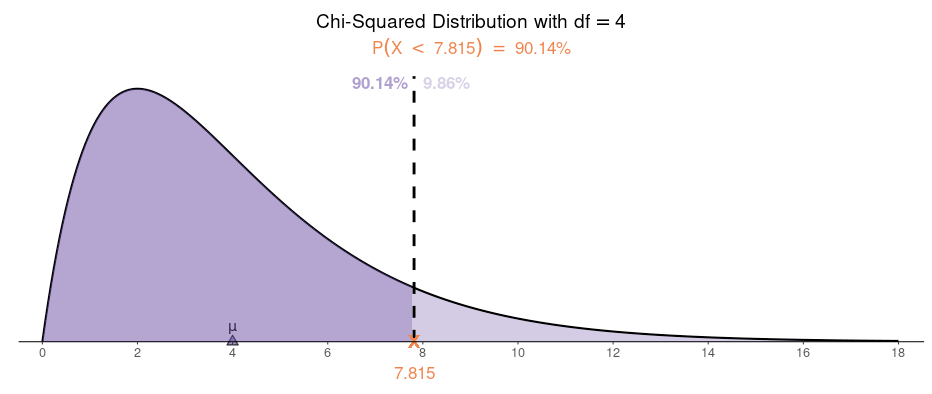See how the shape of the chi-squared distribution depends on the degrees of freedom. Find and visualize probabilities and percentiles (critical values).

• The F Distribution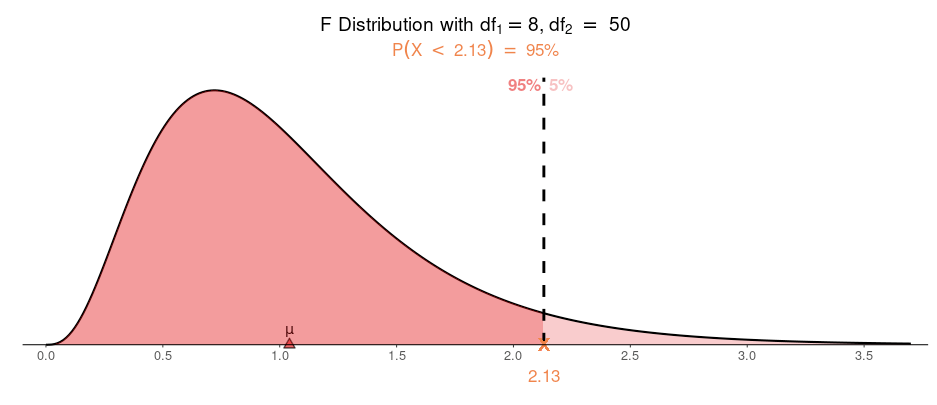See how the shape of the F distribution depends on the degrees of freedom. Find and visualize probabilities and percentiles (critical values).

• The Binomial Distribution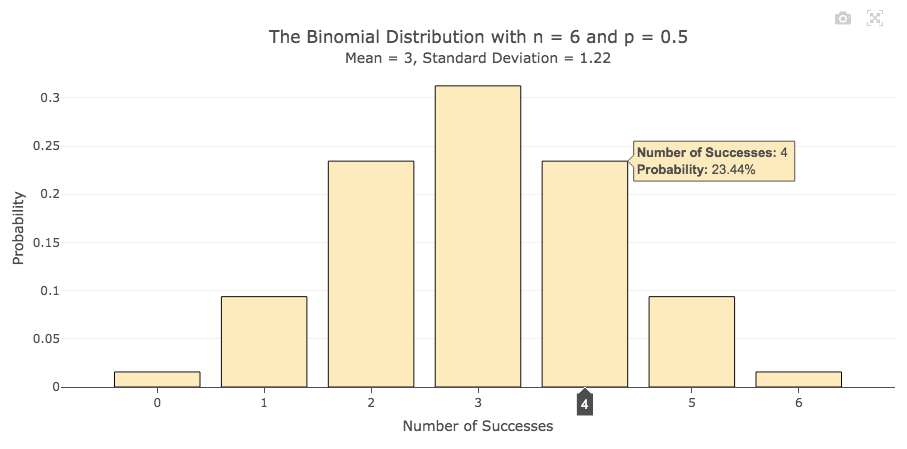Explore how the shape of the binomial distribution depends on the parameter n (the sample size) and p (the probability of success in a Bernoulli trial). Find and visualize probabilities of various kinds.

• The Poisson Distribution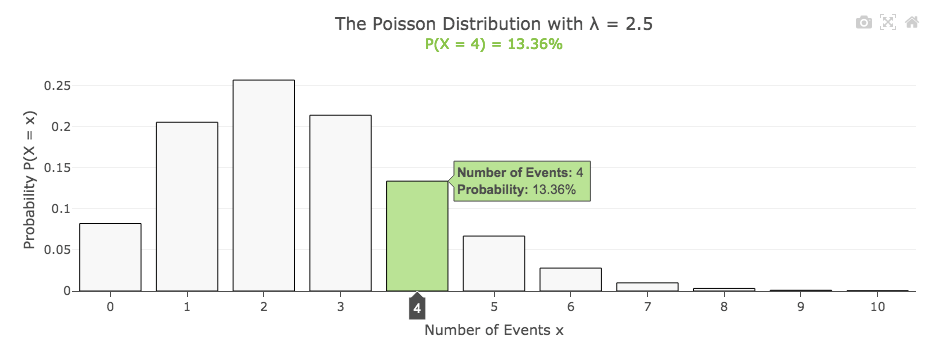Explore how the shape of the Poisson distribution depends on the parameter λ (the mean). Find and visualize various kinds of probabilities.

## Sampling Distributions and the Central Limit Theorem

• Sampling Distribution of the Sample Proportion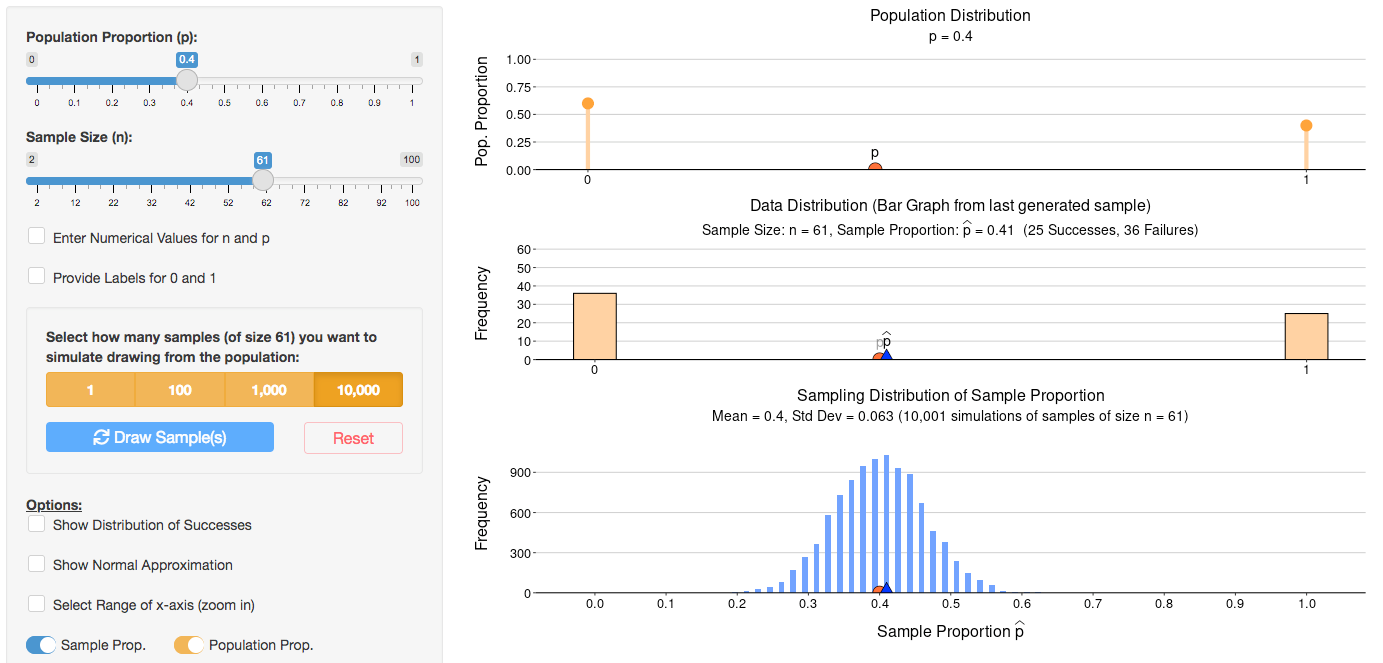Experience how the sampling distribution of the sample proportion builds up one sample at a time. Use sliders to explore the shape of the sampling distribution as the sample size n increases or as the population proportion p changes. Overlay a normal distribution to explore the central limit theorem.

• Sampling Distribution of the Sample Mean (Continuous Population)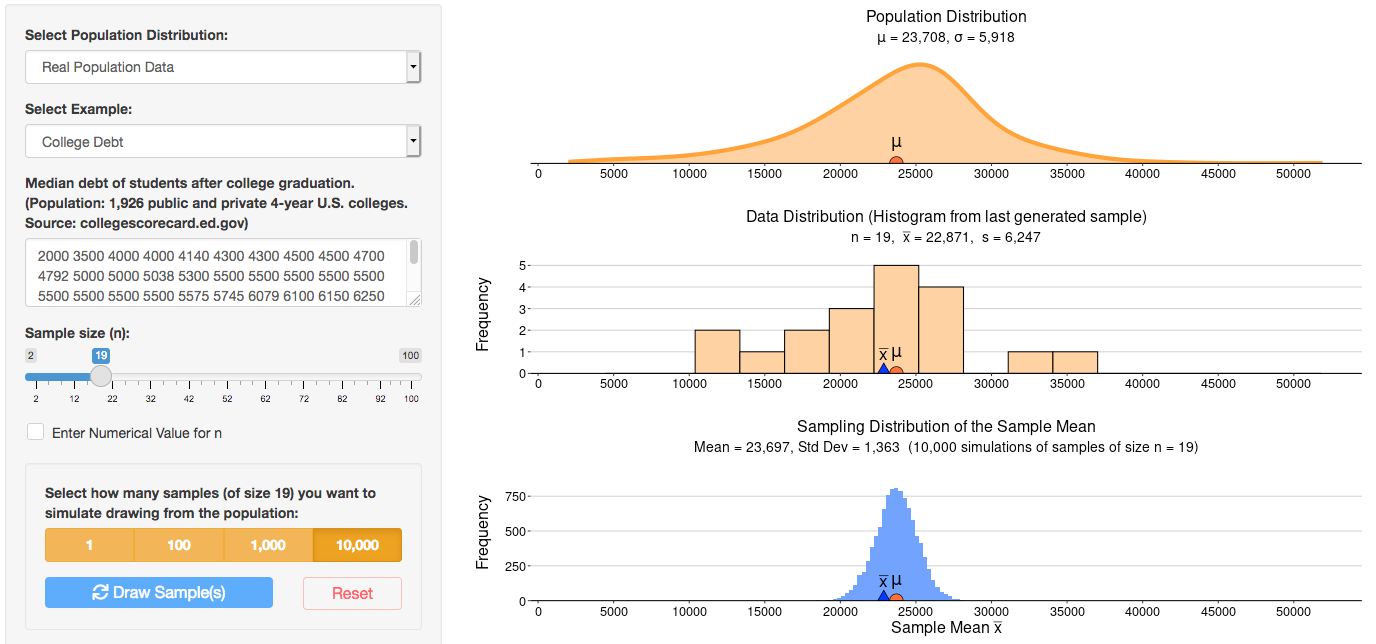Experience how the sampling distribution of the sample mean builds up one sample at a time. Use a variety of real or theoretical continuous population distributions (or create your own) from which to draw samples. Use sliders to gain a deeper understanding of the central limit theorem.

• Sampling Distribution of the Sample Mean (Discrete Population)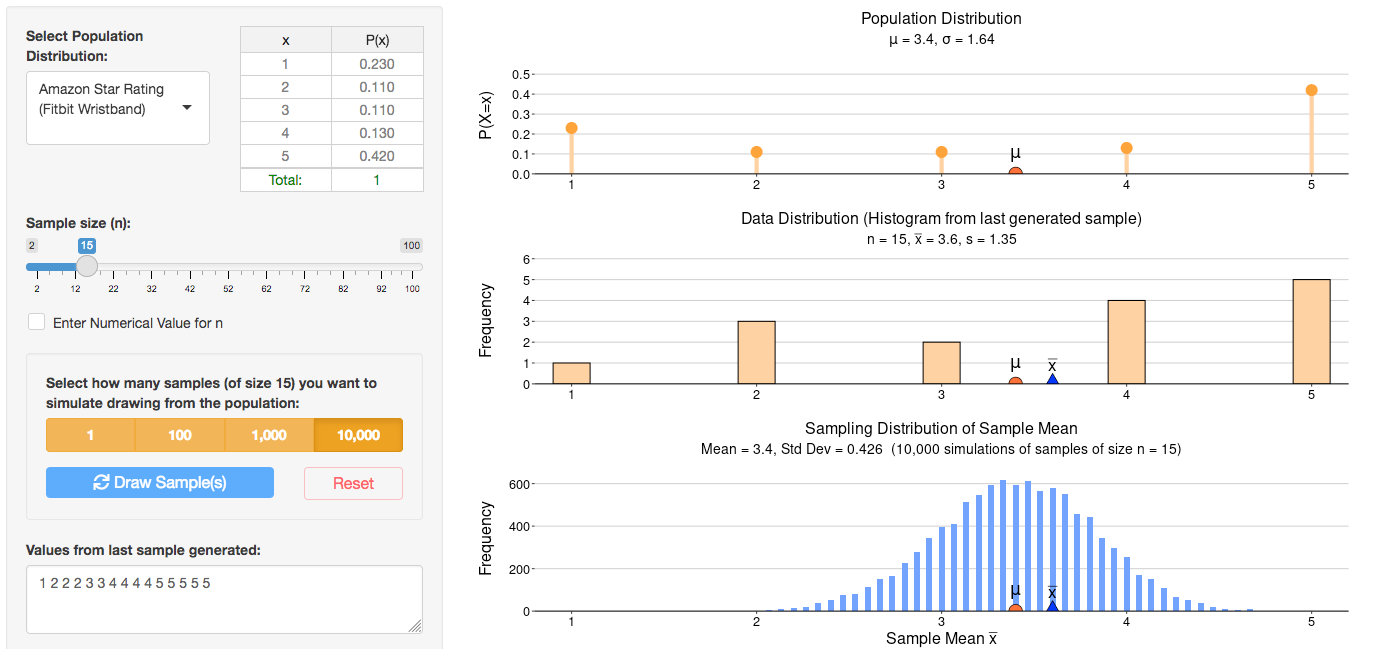Experience how the sampling distribution of the sample mean builds up one sample at a time. Use a variety of real or theoretical discrete population distributions (or create your own) from which to draw samples. Use sliders to gain a deeper understanding of the central limit theorem.

## Confidence Intervals and Significance Tests (One Sample)

• Inference for a Population Proportion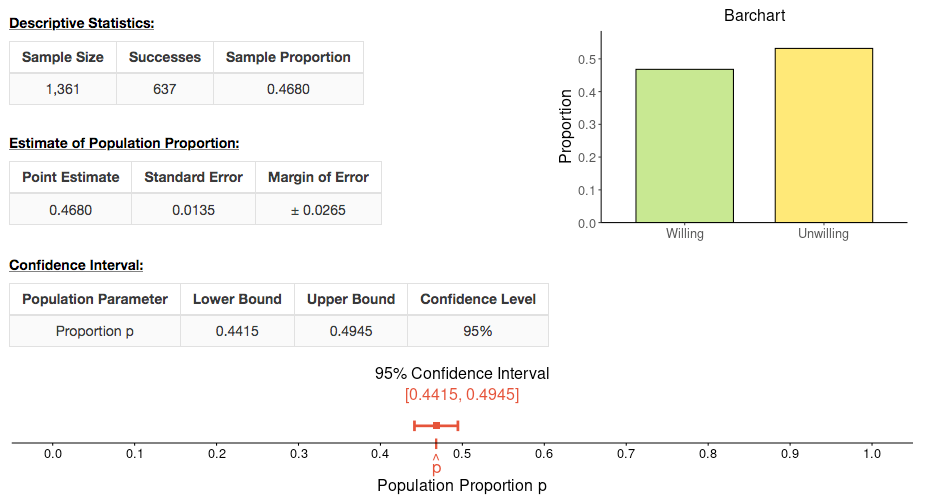Find confidence intervals or test hypotheses about a population proportion. Obtain the margin of error or the z-test statistic and visualize the interval or the P-value on a graph.

• Inference for a Population Mean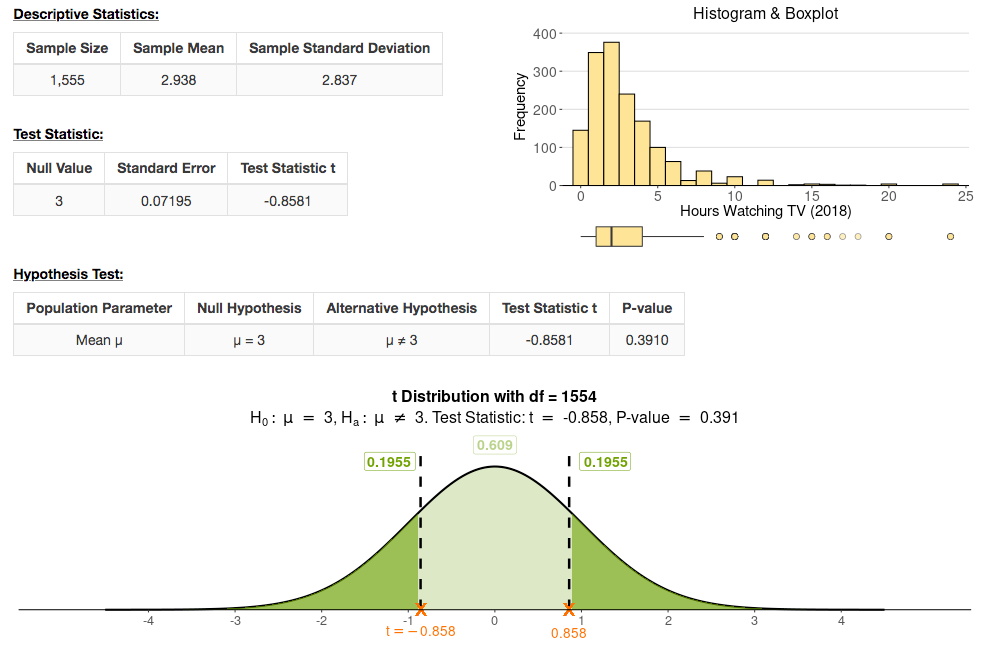Find confidence intervals or test hypotheses about a population mean. Enter your own data or summary statistics. Use plots to check assumptions and visualize the interval or the P-value on a graph.

• Explore Coverage of Confidence Intervals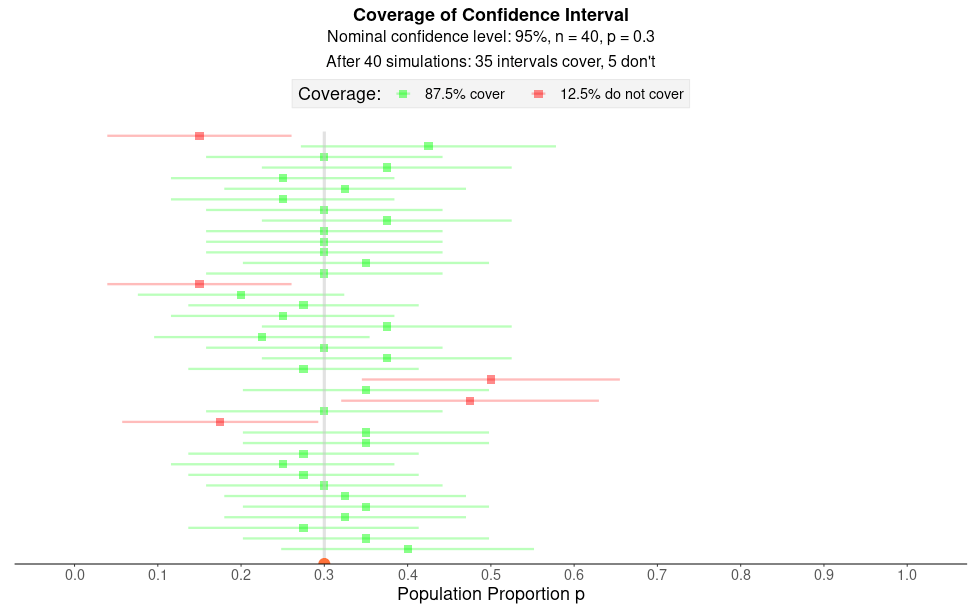What does "95% confidence" mean? What affects the width of an interval? Explore these concepts for confidence intervals of proportions or means, using sliders to change parameters or the sample size.

• Errors and Power in Significance Testing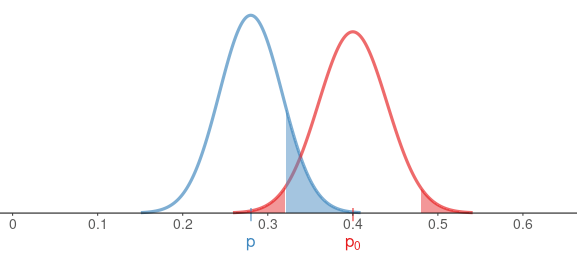Visualize and explore relationships between Type I and Type II errors and the power of a test for proportions or means. See how they depend on sample size and the true values of population parameters.

## Confidence Intervals and Significance Tests (Two Groups)

• Compare Two Population Proportions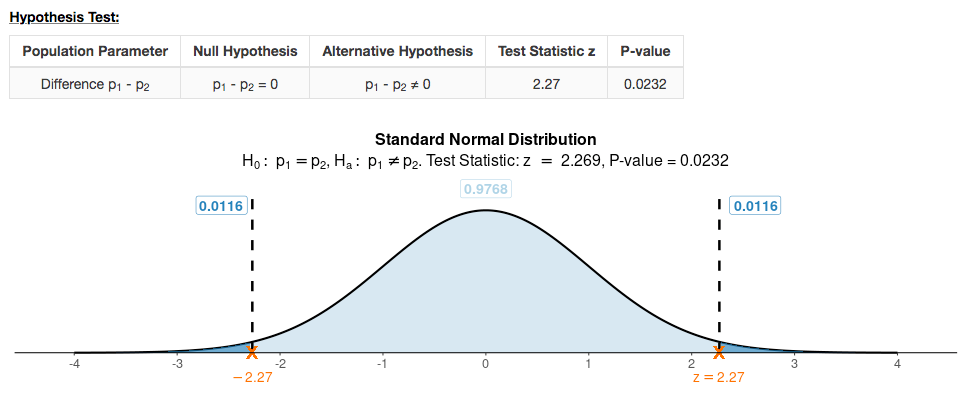Confidence intervals or hypothesis tests about the difference of two population proportions. Obtain the margin of error or the z-test statistic and visualize the interval or the P-value on a graph. For two independent or two dependent samples.

• Compare Two Population Means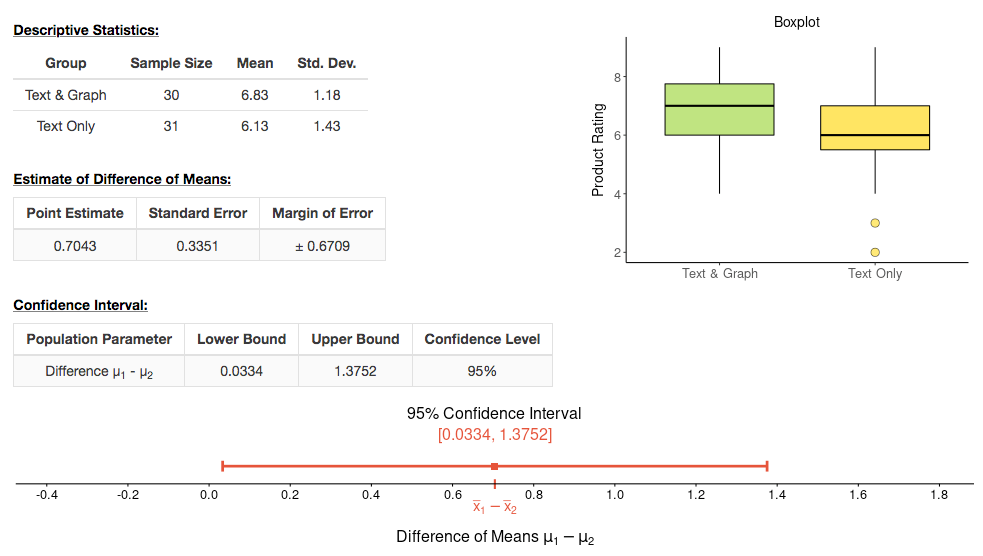Confidence intervals or hypothesis tests about the difference of two population means. Enter your own data or summary statistics. Visualize the interval or the P-value on a graph. For two independent or two dependent samples.

• Fisher’s Exact Test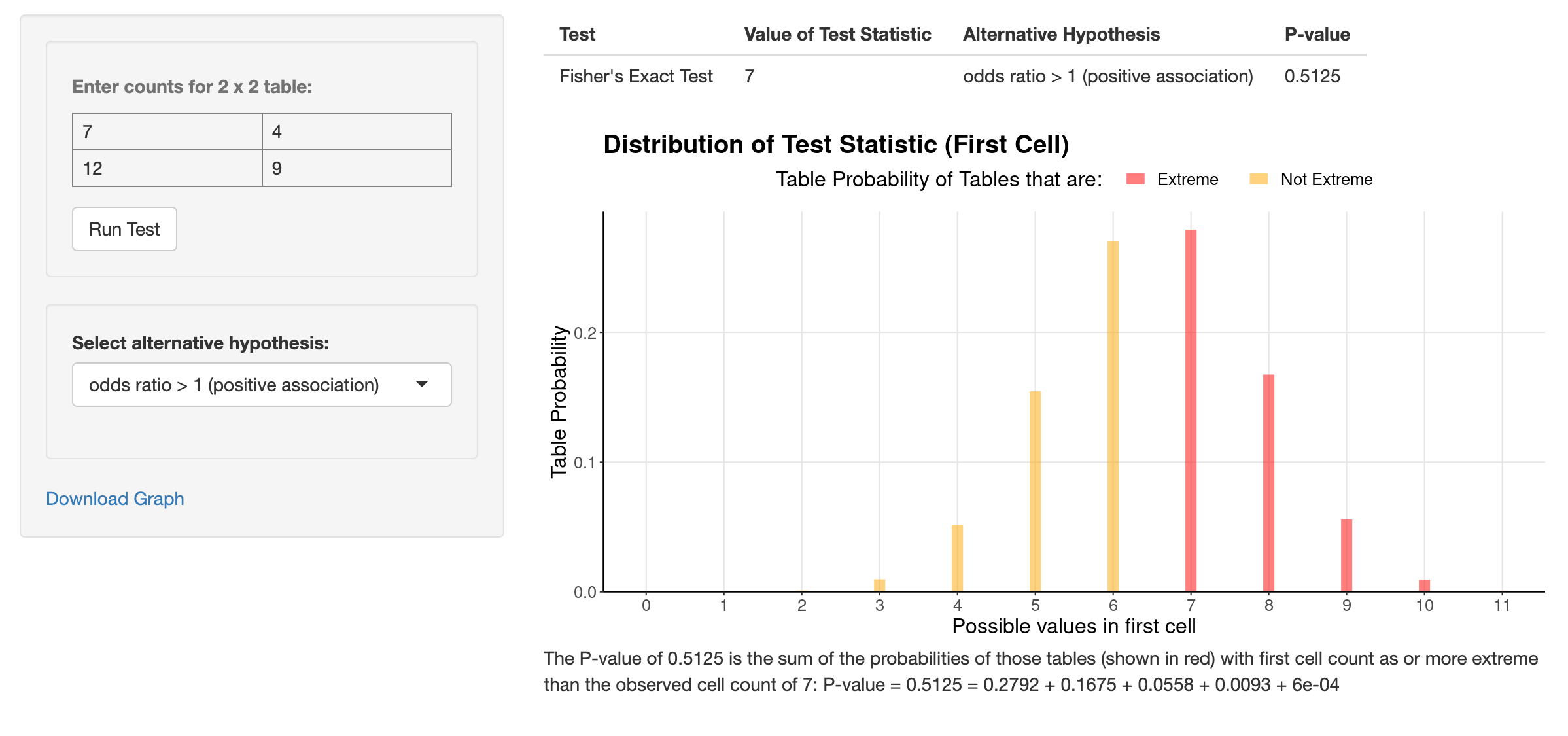Visualize and run Fisher's exact test for 2 x 2 contingency tables. Obtain the exact P-value for one- or two-sided tests.

## Inference for Comparing Several Groups

• The Chi-Squared Test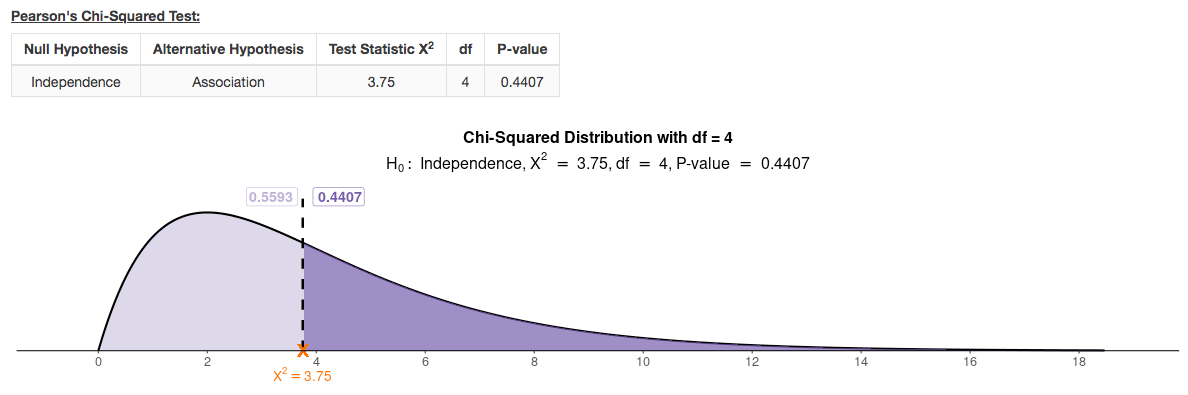Test for independence, homogeneity, or goodness of fit in contingency tables. Enter your own data as raw observations or as a contingency table. Obtain observed and expected counts, and find residuals.

• ANOVA (One-way)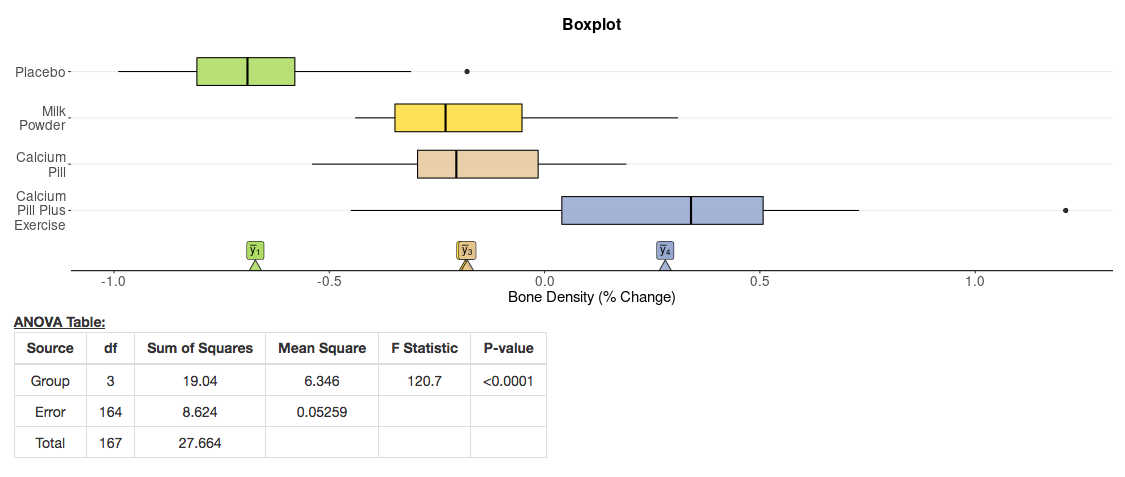Obtain the ANOVA table, F-statistic and side-by-side boxplots to check assumptions. Carry out pairwise comparisons, including simultaneous confidence intervals for pairwise differences of means.